# TExMaT Master Mathematics Teacher 8-12: Limits Chapter Exam

Exam Instructions:

Choose your answers to the questions and click 'Next' to see the next set of questions. You can skip questions if you would like and come back to them later with the yellow "Go To First Skipped Question" button. When you have completed the practice exam, a green submit button will appear. Click it to see your results. Good luck!

### Page 1

#### Question 1 1. Using the graph, find the value of z, then add 1.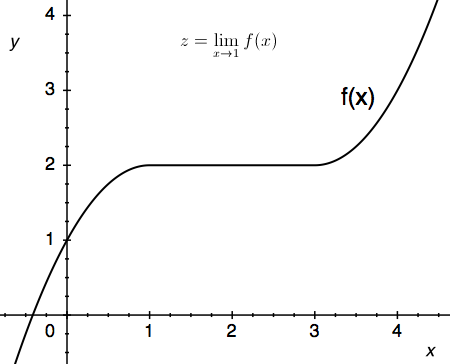#### Question 2 2. Use the given graph and formula to solve for z.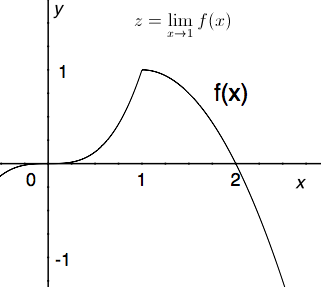#### Question 3 3. Using the given data, solve for the continuous function y.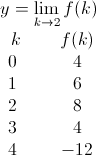#### Question 4 4. Solve for the limit: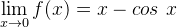#### Question 5 5. Use the given graph and formula to solve for z.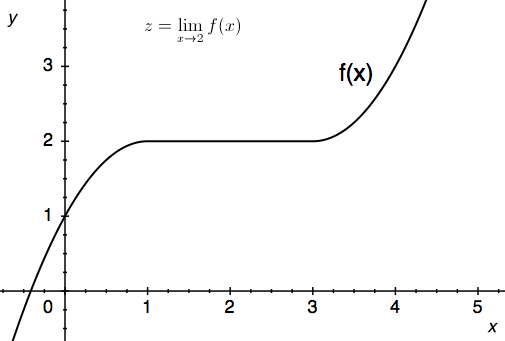### Page 2

#### Question 6 6. The floor function is graphically represented as a stepwise function. What is the limit of f(x) = floor(x) as x approaches 4?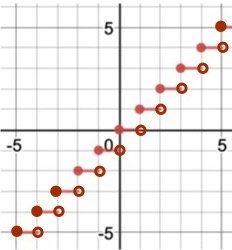#### Question 9 9. Use the graph to solve for z.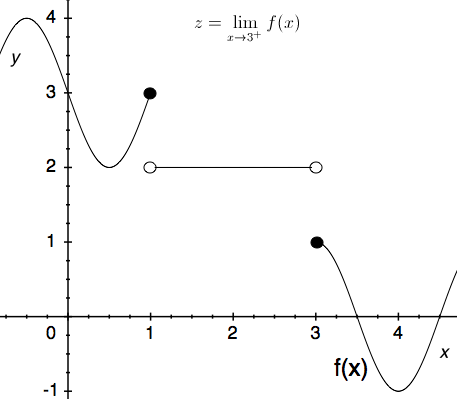### Page 3

#### Question 11 11. Using the given data, predict the likely value of the continuous function z.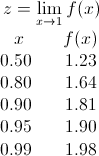#### Question 14 14. Use the graph to solve for z.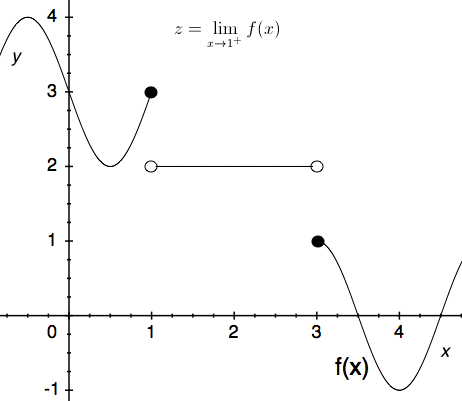### Page 4

#### Question 16 16. Which of the following is true?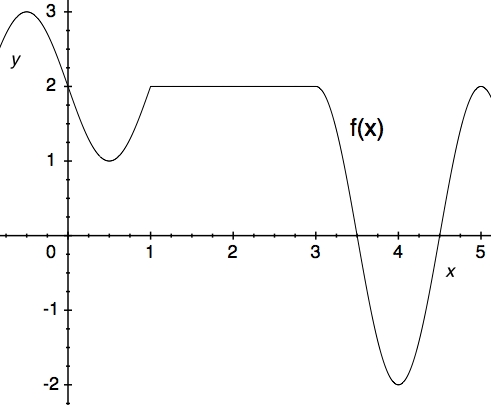#### Question 17 17. Use the given formula to solve for z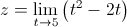#### Question 18 18. Use the graph to solve for z.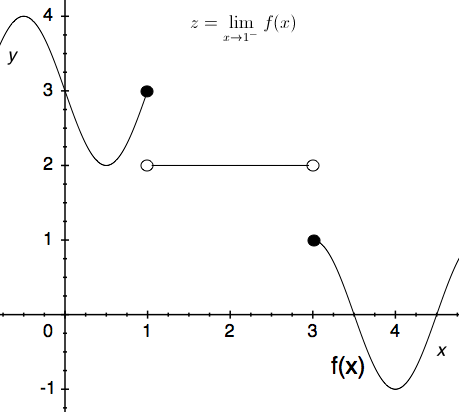#### Question 19 19. Use the graph to solve for z.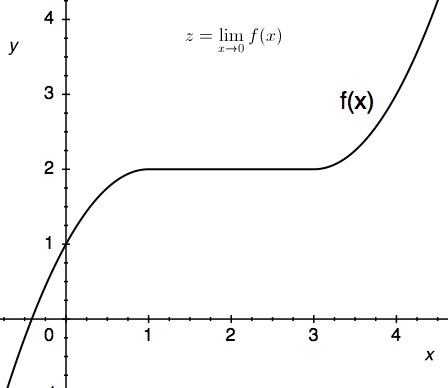#### Question 20 20. The ceiling function is graphically translated as a stepwise function. What is the limit of f(x) = ceil(x) as x approaches 4.5?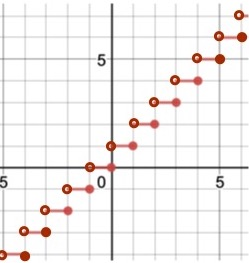#### TExMaT Master Mathematics Teacher 8-12: Limits Chapter Exam Instructions

Choose your answers to the questions and click 'Next' to see the next set of questions. You can skip questions if you would like and come back to them later with the yellow "Go To First Skipped Question" button. When you have completed the practice exam, a green submit button will appear. Click it to see your results. Good luck!

Support